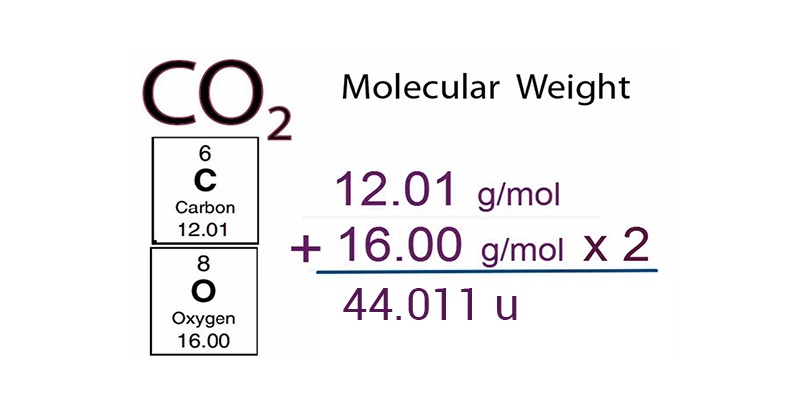Checkout JEE MAINS 2022 Question Paper Analysis : Checkout JEE MAINS 2022 Question Paper Analysis :

# Atomic And Molecular Masses

## Atomic mass

Atoms are very small particles and therefore their masses are also extremely small. Nowadays the method of spectroscopy is used for accurately determining the mass of an atom. But in nineteenth-century scientist used the concept of relative atomic mass for finding the mass of an atom. Elemental hydrogen was considered as the standard and its mass was assumed to be 1 (without any units).Other elements were compared with the atomic mass of hydrogen and their relative masses were obtained. The present scenario is different and now the standard used for atomic masses is carbon 12, an isotope of carbon. This standardization has been accepted all over the globe. The mass of 12C is 12 atomic mass atomic mass units and all the elements are assigned their respective masses according to this standard. One atomic mass unit is equal to

$$\begin{array}{l}\frac{1}{12^{th}}\end{array}$$
of the mass of a carbon-12 atom. The word amu that is atomic mass unit has been replaced by ‘u’ meaning unified mass. If the elements have isotopes then the atomic mass of the element is summation of the relative abundance of the element in multiplication with atomic mass of the respective isotopes.

Other elements were compared with the atomic mass of hydrogen and their relative masses were obtained. The present scenario is different and now the standard used for atomic masses is carbon 12, an isotope of carbon. This standardization has been accepted all over the globe.The mass of 12C is 12 atomic mass units and all the elements are assigned their respective masses according to this standard. One atomic mass unit is equal to

$$\begin{array}{l}\frac{1}{12^{th}}\end{array}$$
of the mass of a carbon-12 atom. The word amu that is atomic mass unit has been replaced by ‘u’ meaning unified mass. If the elements have isotopes then the atomic mass of the element is summation of the relative abundance of the element in multiplication with atomic mass of the respective isotopes.

## Molecular mass

Molecular mass of an element is defined as the sum of the masses of the elements present in the molecule. Molecular mass is obtained by multiplying the atomic mass of an element with the number of atoms in the molecule and then adding the masses of all the elements in the molecule. Let us see an example: carbon dioxide.
The molecular mass of carbon dioxide

$$\begin{array}{l}CO_2\end{array}$$
= atomic mass of carbon + 2 × (atomic mass of oxygen)
= 12.011 u + 2 × (16.00 u)
= 44.011 u
Apart from molecular mass we also have formula mass. This mass is used for compounds which do not have discrete molecules as their constituent units. For example, in NaCl, one Na+ is surrounded by six Cl-and vice versa. The molecular mass in such cases has no meaning since the molecule exists as a single entity.Therefore in these cases we use formula mass for calculating the mass of the molecule.’

Test your knowledge on atomic mass and molecular mass!